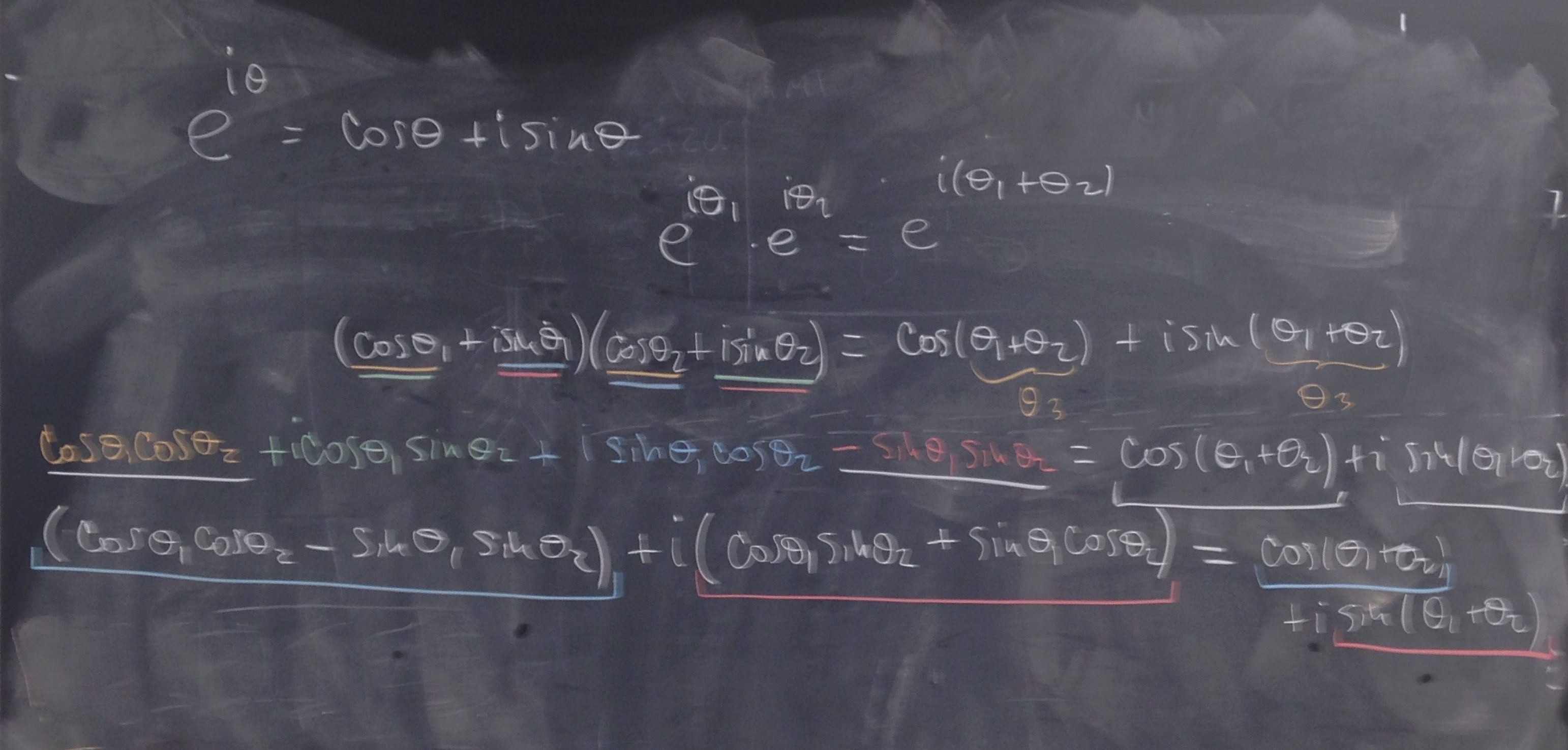For this portion of the course, my key goal is that students understand  how group theory is used in physics, so that they can pursue the subject further if it catches their interest.

In preparation for day 19, students were asked to work through Khan Academy videos on 2×2 matrices outside of class

For most students, this was a review, but some of them have not seen matrices before. Rather than introducing everything about matrices, we only discussed those operations needed to prove that rotation matrices form a group SO(2) under matrix multiplication.

While proving that the set of 2×2 rotation matrices is closed under matrix multiplication, we used our recently acquired knowledge of complex numbers to derive the equations for cosine and sine of the sum of the angles.We introduced SO(3) by analogy to SO(2), but without going into the technical details.

After wrapping up rotations, we then moved on to moving frames of reference and the Galilean principle of relativity. We showed that acceleration is the same in all frames of reference moving at constant velocity relative to one another. This calculation required the understanding of a derivative, which was familiar to most students but not all, so I emphasized the conceptual understanding. We then defined Galilean group.